# A Simple French Curve Emulator

## Preamble

A French Curve is a device used in hand-crafting technical figures to draw a smooth curve through an ordered series of fixed points in the plane. The purpose is purely aesthetic, with no claim for the result to have any optimality property.

In R the function stats::spline is often adequate to draw a smooth interpolation curve between fixed points, but this is restricted to cases where the points are ordered so that their $$x-$$coordinates are monotonic. If this is not the case, and the required curve “doubles back” on itself, then an alternative method is needed. An extension of this is the case when the interpolation curve is required to be closed, that is, when it has to loop around from the last point back to the first in a continuous smooth fashion.

## Example

An example is useful to fix ideas. We define five points in the plane by the following data.frame. In the figure, the arrows indicate the ordering.

pts <- data.frame(x = c(0.286, 0.730, 0.861, 0.623, 0.100),
y = c(0.164, 0.206, 0.514, 0.666, 0.492))
with(pts, {
par(mar = c(4, 4, 1, 1), las = 1)
plot(x, y, asp = 1, col = 4, panel.first = grid(), pch = 1, cex = 2, bty = "n")
arrows(x[-5], y[-5], x[-1], y[-1], angle = 15, length = 0.125, col = 2)
})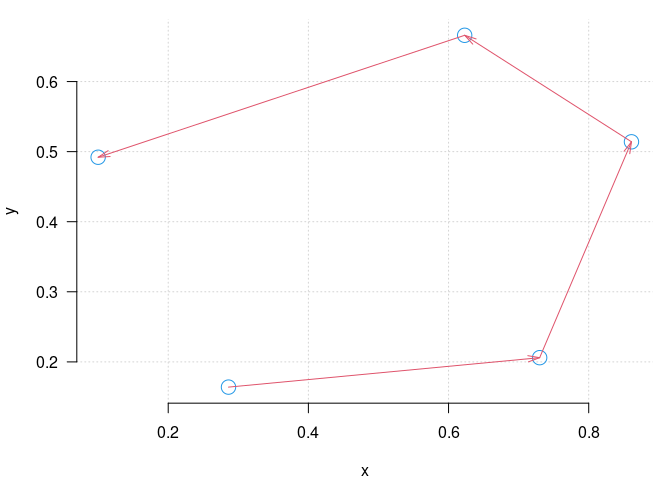Clearly stats::spline cannot be used directly to produce an interpolating curve in this case as neither $$x-$$ or $$y-$$coordinates are monotonically ordered.

The simple solution we offer here is to use use arc length along the line segments joining the points as a parameter and fit interpolating splines to both $$x-$$ and $$y-$$ coordinates of the given points as a function of arc length.

More explicitly, we take the cumulative Euclidean distance lengths of the arrow segments in the diagram above as the parameter and fit interpolating splines to the $$x-$$ and $$y-$$ coordinates of the lengths separately, and use the splines as the coordinates of the interpolating curve. The method is shown in the code below.

s <- with(pts, cumsum(c(0, sqrt(diff(x)^2 + diff(y)^2))))
icurve <- with(pts, data.frame(x = spline(s, x, n = 500)$y, y = spline(s, y, n = 500)$y))
with(pts, {
par(mar = c(4, 4, 1, 1), las = 1)
with(icurve, plot(x, y, asp = 1, panel.first = grid(), type = "l", bty = "n", col = 2))
points(x, y)
})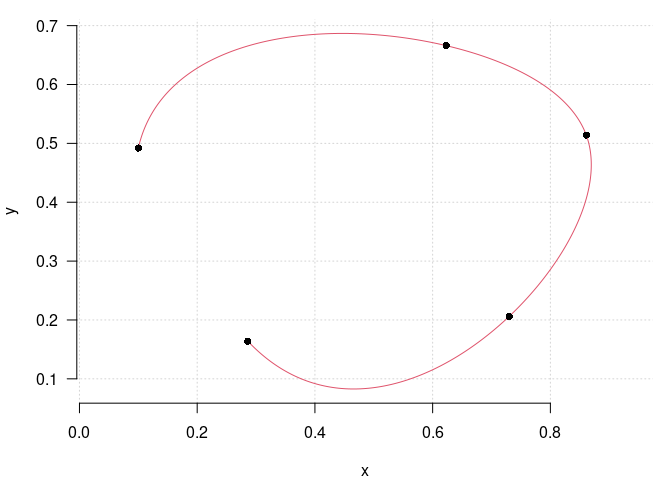This is essentially the operation of the function frenchCurve::open_curve. The function produces an S3 object with class "curve" for which several methods are available, including its own plot and lines methods for traditional graphics.

### Scale dependence

One further tweak is provided by the two main functions of the package. The Euclidean distances used in the computation are critically dependent on the relative scales of the $$x-$$ and $$y-$$coordinates. It is up to the user to use the functions with the coordinates scaled in such a way as to make the Euclidean distance the appropriate metric. To help with this, both functions frenchCurve::open_curve and frenchCurve::closed_curve provide an argument asp to specify a scale adjustment. Specifically, the two coordinates x and y * asp are used in the distance computations for arc length.

The asp argument is a single numerical value with a default of 1. However it may be supplied as a character string and asp = "range" specifies that the value asp = diff(range(x))/diff(range(y)) should be used. The effect is shown in the following extension to the running example below.

icurve <- open_curve(pts)
jcurve <- open_curve(pts, asp = "range")
plot(icurve, bty = "n", col = 2, asp = 1)
grid()
lines(jcurve, col = 4)
legend("topright", legend = c("asp = 1", 'asp = "range"'),
lty = "solid", col = c(2,4), pch=20, bty = "n", cex = 0.75)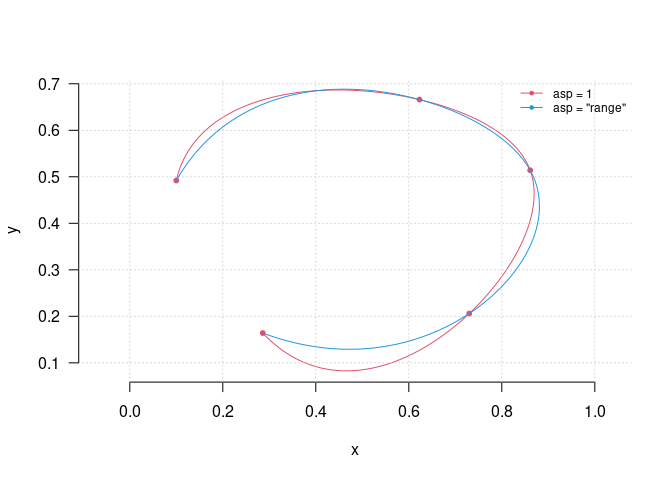Notice particularly that the asp argument to open_curve and the asp argument to graphics::plot are different, but have a similar purpose.

### Closed curves

If the curve is required to link back from the last point to the first in a smooth continuous way, the algorithm we propose is simply to repeat the points three times and choose the middle section of the result. This may be overkill, but the computation is relatively cheap and the result usually appears satisfactory for most aesthetic purposes.

The results for the running example are shown in the figure below:

iccurve <- closed_curve(pts)
jccurve <- closed_curve(pts, asp = "range")
plot(iccurve, bty = "n", col = 2, asp = 1)
grid()
lines(jccurve, col = 4)
legend("topright", legend = c("asp = 1", 'asp = "range"'),
lty = "solid", col = c(2,4), pch=20, bty = "n", cex = 0.75)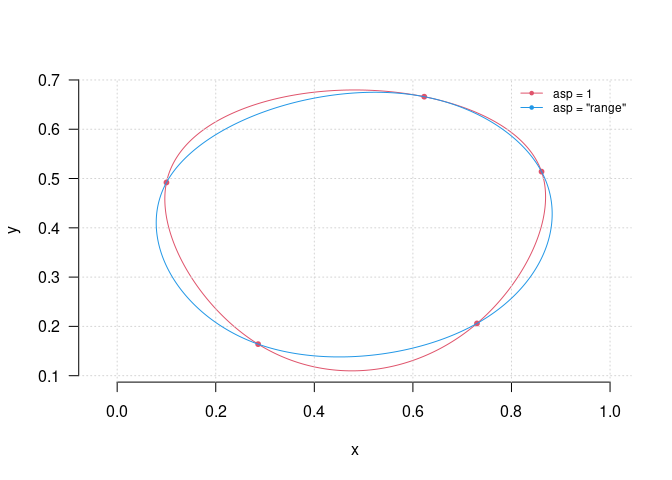## Other tools

The package also provides a similar facility for Bezier curve interpolation using the given points as the control points.

An often forgotten feature of traditional graphics is that it can use complex vectors to specify points. Complex vectors are also very useful for the computations needed here. The following example shows a few of these features.

set.seed(2345)
z <- (complex(argument = seq(-0.9*base::pi, 0.9*base::pi, length = 20)) +
complex(modulus = 0.125, argument = runif(20, -base::pi, base::pi))) *
complex(argument = runif(1, -base::pi, base::pi))

par(pty = "s", mfrow = c(2, 2), mar = c(1,1,2,1))
plot(z, asp = 1, axes = FALSE, ann = FALSE, panel.first = grid())
title(main = "Open")
segments(Re(z), Im(z), Re(z), Im(z), col = "grey", lty = "dashed")
lines(open_curve(z), col = "red")

plot(z, asp = 1, axes = FALSE, ann = FALSE, panel.first = grid())
title(main = "Closed")
lines(closed_curve(z), col = "royal blue")

plot(z, asp = 1, axes = FALSE, ann = FALSE, panel.first = grid())
title(main = "Bezier")
lines(bezier_curve(z), col = "dark green")

plot(z, asp = 1, axes = FALSE, ann = FALSE, panel.first = grid())
title(main = "Circle")
lines(complex(argument = seq(-base::pi, base::pi, len = 500)),
col = "purple")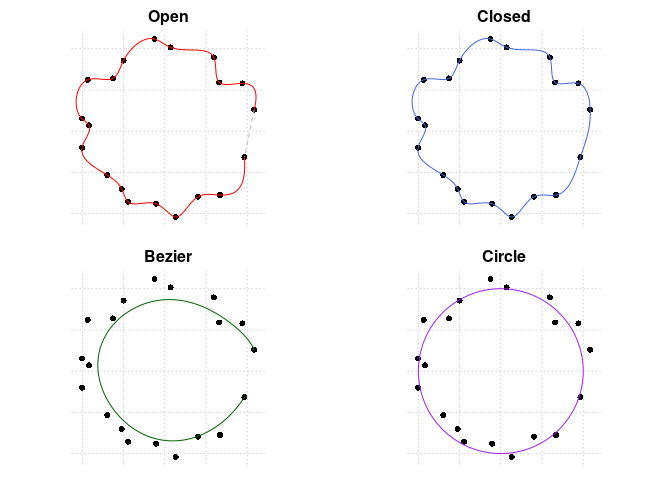### grid-based graphics systems

The package is set up to use traditional graphics by default, but the changes necessary to use grid-bases systems such as ggplot2 or lattice graphics are minor and obvious. We illustrate this in the example below.

library(ggplot2)
set.seed(1234)
z <- complex(real = runif(5), imaginary = runif(5))
z <- z[order(Arg(z - mean(z)))]
cz <- closed_curve(z)
oz <- open_curve(z)
ggplot(as.data.frame(z)) +
geom_path(data = as.data.frame(cz), aes(x,y), colour = "#DF536B") +
geom_path(data = as.data.frame(oz), aes(x,y), colour = "#2297E6") +
geom_point(aes(x = Re(z), y = Im(z))) +
geom_segment(aes(x    = Re(mean(z)), y    = Im(mean(z)),
xend = Re(z),       yend = Im(z)),
arrow = arrow(angle=15, length=unit(0.125, "inches")),
colour = alpha("grey", 2/3)) + coord_equal() +
theme_bw()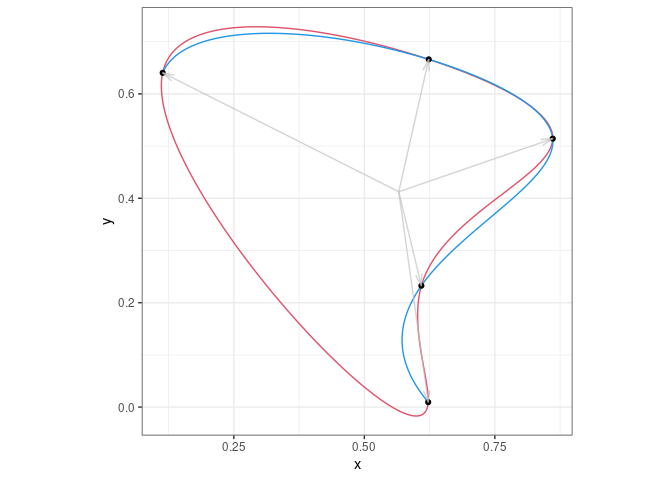Notice that the $$x-$$ and $$y-$$coordinates may be specified for the two main functions in any form accepted by the traditional graphics plotting functions, as handled by the auxiliary function grDevices::xy.coords. These are

• As two separate numeric vectors x and y,
• As a list or data.frame with two of its components numeric vectors names "x" and "y",
• As a two-column numeric matrix, with the first column understood to be x, and
• As a complex vector.

The main tool supplied in the package for linking with other graphics systems is as.data.frame.curve which allows objects inheriting from class "curve" to be seamlessly converted to data.frames. The function base::as.data.frame.complex is already provided, but is less useful for our purposes here.

## Postamble

The only justification I have for this package is that I have found it useful in my own work on several occasions, mostly unexpectedly. It has been handy to have the computations, simple as they are, packaged and easily available for my use. I hope it proves useful for others as well.